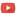KE8QZC | SFW | TSWSyllabus
Student calendar
Test 1 formula sheet

Quizzes
Quiz 1 (solution)
Quiz 2 (solution)
Quiz 3 (solution)

Mathematica notebooks
Notebook from 24 Sept 2015 -- functions of 2 variables, level curves
Notebook from 25 Sept 2015 -- functions of 2 variables, complex graph colorings
Notebook from 8 October 2015 -- minima and maxima